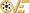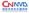# Apple Mac OS X inet_net_pton API 整形溢出漏洞

 漏洞ID 1184303 漏洞类型 数字错误 发布时间 2008-12-15 更新时间 2008-12-17CVE编号 CVE-2008-4220CNNVD-ID CNNVD-200812-298 漏洞平台 N/A CVSS评分 10.0
##### |漏洞详情
MacOSX是苹果家族机器所使用的操作系统。AppleMacOSX10.5.6以前的版本中inet_net_ptonAPI存在整形溢出漏洞，这允许视情况而定的攻击者通过未明的攻击方式来执行任意的代码或导致拒绝服务。注意：这可能与SecurityReason在20080822发布的WLB-2008080064公告有关，因此和20081216一样，因为细节不足，无法确定。
##### |漏洞EXP
``````[WLB-2008080064: inet_net_pton() integer overflow ]

Author: Maksymilian Arciemowicz
Date:
- - Written: 02.08.2008
- - Public: 22.08.2008

Risk: Low
It is a bug, without a high security risk. We are going informing all vendors, about this problem.

Affected Software:
libc inet_net_pton.c
ver ISC Bind
- - OpenBSD fixed

Vendor: http://www.isc.org/index.pl?/sw/bind/index.php

- --- 0.Description ---
inet_net_pton - Internet network number manipulation routines

SYNOPSIS:
int
inet_net_pton(int af, const char *src, void *dst, size_t size);

The inet_net_pton() function converts a presentation format Internet network number (that is, printable form as held in a character string) to network format (usually a struct in_addr or some other internal binary representation, in network byte order).  It returns the number of bits (either computed based on the class, or specified with /CIDR), or -1 if a failure occurred (in which case errno will have been set.  It will be set to ENOENT if the Internet network number was not valid).

Caution: The dst field should be zeroed before calling inet_net_pton() as the function will only fill the number of bytes necessary to encode the network number in network byte order.

The only value for af currently supported is AF_INET.  size is the size of the result buffer dst.

NETWORK NUMBERS (IP VERSION 4)
The external representation of Internet network numbers may be specified in one of the following forms:

a
a.b
a.b.c
a.b.c.d

Any of the above four forms may have ``/bits'' appended where ``bits'' is in the range 0-32 and is used to explicitly specify the number of bits in the network address.  When ``/bits'' is not specified the number of bits

- --- 1. libc/net inet_net_pton() integer overflow ---
The main problem exist in inet_net_pton() function. Let's see to this function

inet_net_pton.c

- ---
int
inet_net_pton(int af, const char *src, void *dst, size_t size)
{
switch (af) {
case AF_INET:
return (inet_net_pton_ipv4(src, dst, size));
default:
errno = EAFNOSUPPORT;
return (-1);
}
}
- ---

call to inet_net_pton_ipv4(). So let's see it..

- -START--
static int
inet_net_pton_ipv4(const char *src, u_char *dst, size_t size)
{
static const char
xdigits[] = "0123456789abcdef",
digits[] = "0123456789";
int n, ch, tmp, dirty, bits;
const u_char *odst = dst;

ch = *src++;
if (ch == '0' && (src == 'x' || src == 'X')
&& isascii(src) && isxdigit(src)) {
/* Hexadecimal: Eat nybble string. */
if (size <= 0)
goto emsgsize;
*dst = 0, dirty = 0;
src++;	/* skip x or X. */
while ((ch = *src++) != '\0' &&
isascii(ch) && isxdigit(ch)) {
if (isupper(ch))
ch = tolower(ch);
n = strchr(xdigits, ch) - xdigits;
assert(n >= 0 && n <= 15);
*dst |= n;
if (!dirty++)
*dst <<= 4;
else if (size-- > 0)
*++dst = 0, dirty = 0;
else
goto emsgsize;
}
if (dirty)
size--;
} else if (isascii(ch) && isdigit(ch)) {
/* Decimal: eat dotted digit string. */
for (;;) {
tmp = 0;
do {
n = strchr(digits, ch) - digits;
assert(n >= 0 && n <= 9);
tmp *= 10;
tmp += n;
if (tmp > 255)
goto enoent;
} while ((ch = *src++) != '\0' &&
isascii(ch) && isdigit(ch));
if (size-- <= 0)
goto emsgsize;
*dst++ = (u_char) tmp;
if (ch == '\0' || ch == '/')
break;
if (ch != '.')
goto enoent;
ch = *src++;
if (!isascii(ch) || !isdigit(ch))
goto enoent;
}
} else
goto enoent;

bits = -1;
if (ch == '/' && isascii(src) && isdigit(src) && dst > odst) {
/* CIDR width specifier.  Nothing can follow it. */
ch = *src++;	/* Skip over the /. */
bits = 0;
do {
n = strchr(digits, ch) - digits;
assert(n >= 0 && n <= 9);
bits *= 10;
bits += n;
} while ((ch = *src++) != '\0' &&
isascii(ch) && isdigit(ch));
- -SLEEP---

bits integer is signed,

- -WAKEUP---
if (ch != '\0')
goto enoent;
if (bits > 32)
goto emsgsize;
- -SLEEP---

if bits > 32 , we will go to emsgize,
but for
*dst = "127.0.0.1/2147483649"

<=>

bits=-2147483647

we will not goto emsgsize

ok, continue

- --WAKEUP---
}

/* Firey death and destruction unless we prefetched EOS. */
if (ch != '\0')
goto enoent;

/* If nothing was written to the destination, we found no address. */
if (dst == odst)
goto enoent;
/* If no CIDR spec was given, infer width from net class. */
if (bits == -1) {
if (*odst >= 240)	/* Class E */
bits = 32;
else if (*odst >= 224)	/* Class D */
bits = 4;
else if (*odst >= 192)	/* Class C */
bits = 24;
else if (*odst >= 128)	/* Class B */
bits = 16;
else			/* Class A */
bits = 8;
/* If imputed mask is narrower than specified octets, widen. */
if (bits < ((dst - odst) * 8))
bits = (dst - odst) * 8;
}
/* Extend network to cover the actual mask. */
while (bits > ((dst - odst) * 8)) {
if (size-- <= 0)
goto emsgsize;
*dst++ = '\0';
}
return (bits);
- -STOP---
...
- -END---

(bits > ((dst - odst) * 8))==FALSE

so '\0' will not be set in *dst.

bits is returned.

For example:
af=AF_INET
src=127.0.0.1/2147483649

function will return -2147483647
and pointer dst will don't have null byte of end.

A lot of programs use inet_net_pton() in if() function, like

if(inet_net_pton(...)!=-1){
blabla...
} else ERROR...

In specification we can find
- ---
...It returns the number of bits (either computed based on the class, or specified with /CIDR), or -1 if a failure occurred (in which case errno will have been set...
- ---
/* Only -1 is reserved for errors. */

and

- ---
Any of the above four forms may have ``/bits'' appended where ``bits'' is in the range 0-32 and is used to explicitly specify the number of bits in the network address.  When ``/bits'' is not specified the number of bits
#include <arpa/inet.h>
- ---

so
if (bits > 32)
goto emsgsize;

dosen't protect us, before integer overflow.

Security Risk is here very low, but bug should be corrected. We will only inform all vendors.
ISC BIND has been informed and confirmed existing a bug.

- ---
...
I don't see this as something one needs to be alarmed about. It is a bug and it does need to be addressed. Vendors that have included our code do need to be informed.
...
- ---

- ---
...
It just looks like a bug that for now that has, luckily, no security consequences, as far as we can see
...
- ---

- ---
...
Additionally dst is a binary blob, not a C string, and as such doesn't
require '\0' termination.
While returning the wrong value for when the cidr mask length overflows
is a bad, almost all inputs to this function will be from configuration
files. For this to be a issue there needs to be gross configuration
errors in those configuration files.
...
- ---

- --- 2. Credit ---
Maksymilian Arciemowicz``````
##### |受影响的产品
Apple Mac OS X Server 10.5.5 Apple Mac OS X Server 10.5.4 Apple Mac OS X Server 10.5.3 Apple Mac OS X Server 10.5.2 Apple Mac OS X Server 10.5.1 Apple Mac OS X Server 10.